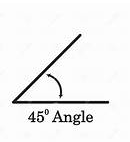# McGraw Hill Math Grade 4 Chapter 13 Lesson 1 Answer Key Angles and Circles

Practice the questions of McGraw Hill Math Grade 4 Answer Key PDF Chapter 13 Lesson 1 Angles and Circles to secure good marks & knowledge in the exams.

## McGraw-Hill Math Grade 4 Answer Key Chapter 13 Lesson 1 Angles and Circles

Solve

Write each missing number.

Question 1.
1 right angle = ___________
90

Explanation:
1 right angle measures 90 degrees.

Question 2.
2 right angle = ___________
180

Explanation:
1 right angle = 90
2 right angles = 2 x 90 = 180
So, 2 right angles = 180 degrees.

Question 3.
3 right angle = ___________
270

Explanation:
1 right angle = 90
3 right angles = 3 x 90 = 270
So, 3 right angles = 270 degrees.

Question 4.
4 right angle = ___________
1 right angle = 90
4 right angles = 4 x 90 = 360
So, 4 right angles = 360 degrees.

Question 5.
A 90-degree angle is a right angle. What would a 45-degree angle look like? Draw it.